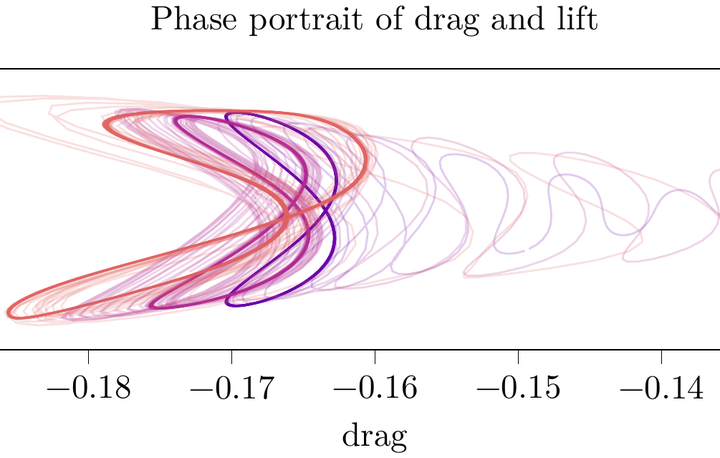# Convolutional Neural Networks for Very Low-Dimensional LPV Approximations of Incompressible Navier-Stokes Equations### Abstract

The control of general nonlinear systems is a challenging task in particular for large-scale models as they occur in the semi-discretization of partial differential equations (PDEs) of, say, fluid flow. In order to employ powerful methods from linear numerical algebra and linear control theory, one may embed the nonlinear system in the class of linear parameter varying (LPV) systems. In this work, we show how convolutional neural networks can be used to design LPV approximations of incompressible Navier-Stokes equations. In view of a possibly low-dimensional approximation of the parametrization, we discuss the use of deep neural networks (DNNs) in a semi-discrete PDE context and compare their performance to an approach based on proper orthogonal decomposition (POD). For a streamlined training of DNNs directed to the PDEs in a Finite Element (FEM) framework, we also discuss algorithmical details of implementing the proper norms in general loss functions.

Type
Publication
Frontiers Appl. Math. Stat.